# RS Aggarwal Solutions for Class 7 Maths Exercise 3C Chapter 3 Decimals

RS Aggarwal Solutions for Class 7 Maths Exercise 3C Chapter 3 Decimals in simple PDF are given here. This exercise of RS Aggarwal Solutions for Class 7 Chapter 3 contains questions related to multiplication of decimals by 10, 100, 100 etc., multiplication of a decimal by a whole number and multiplication of a decimal by a decimal. On multiplying a decimal by 10, 100, 1000, the decimal point is shifted to the right by one place, two places and three places respectively. Learn more about these topics by solving the questions of RS Aggarwal Solutions for Class 7 Maths Chapter 3 Decimals Exercise 3C with the help of solutions provided here.

## Download the PDF of RS Aggarwal Solutions For Class 7 Maths Chapter 3 Decimals – Exercise 3C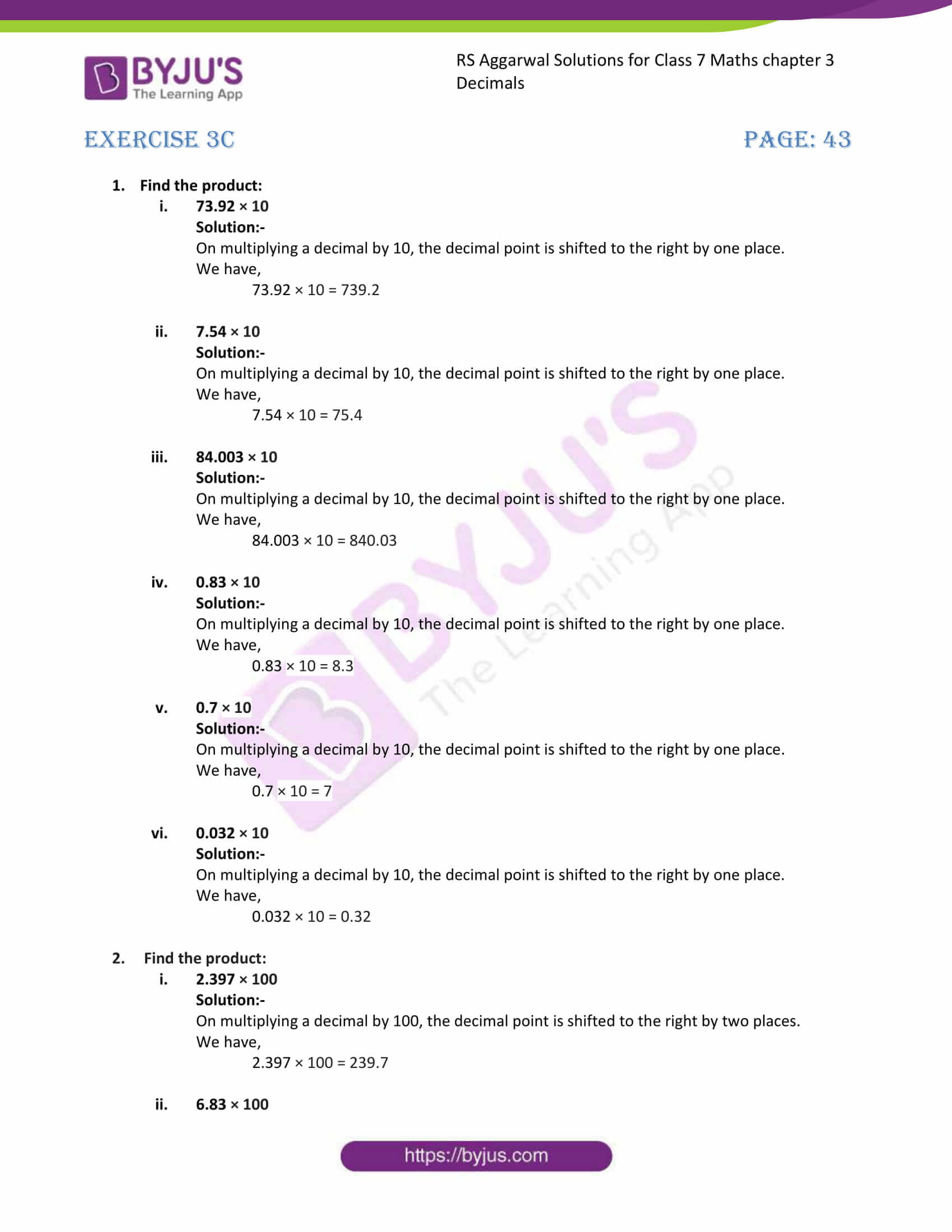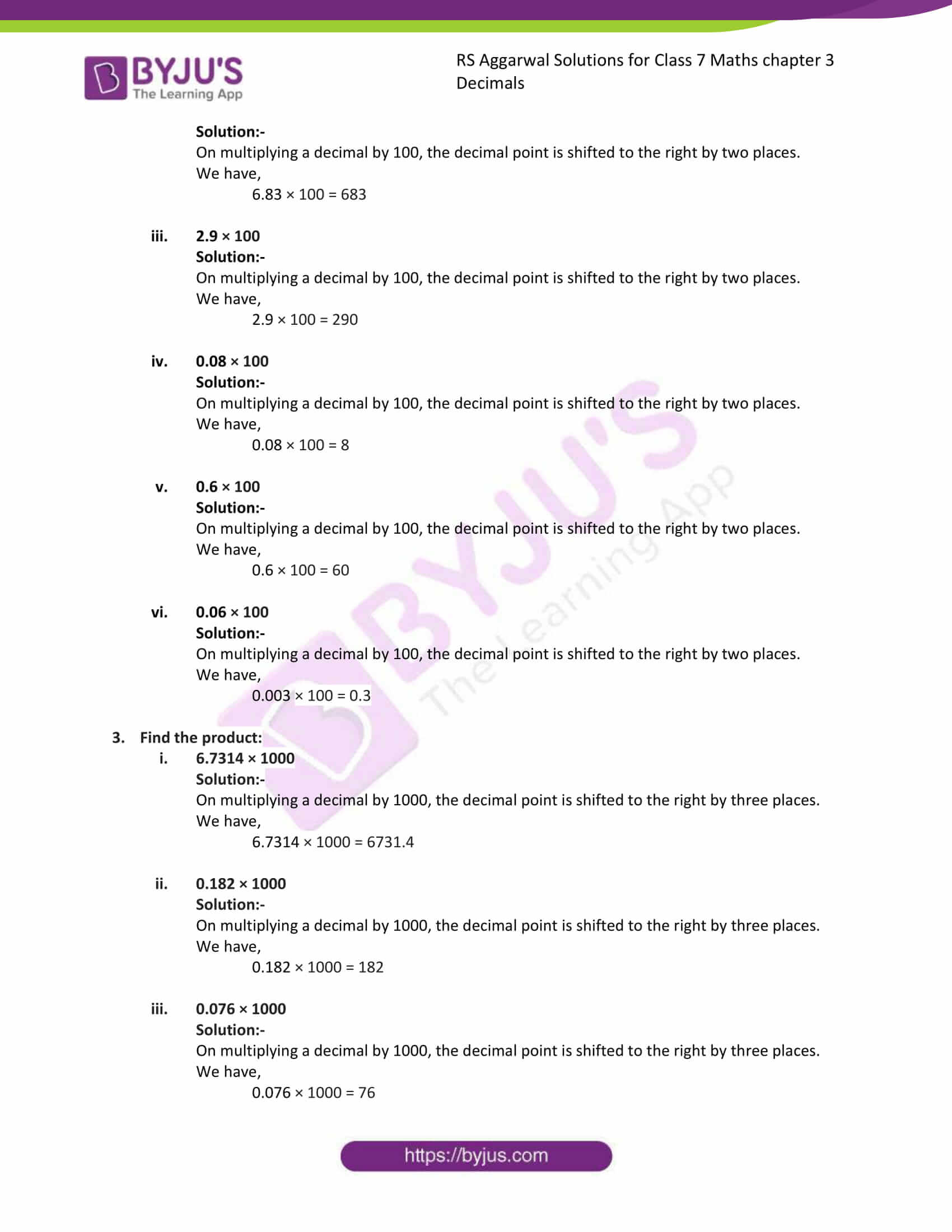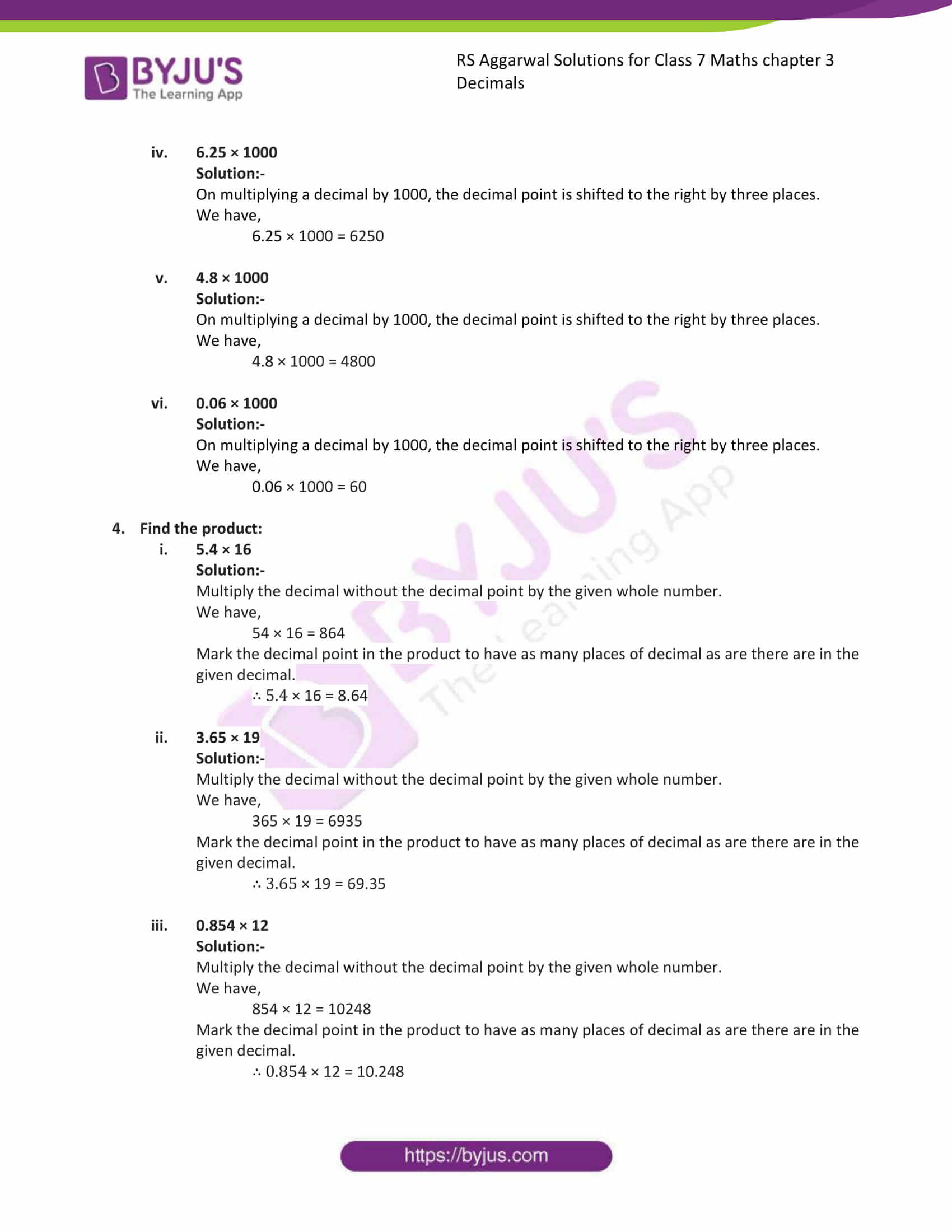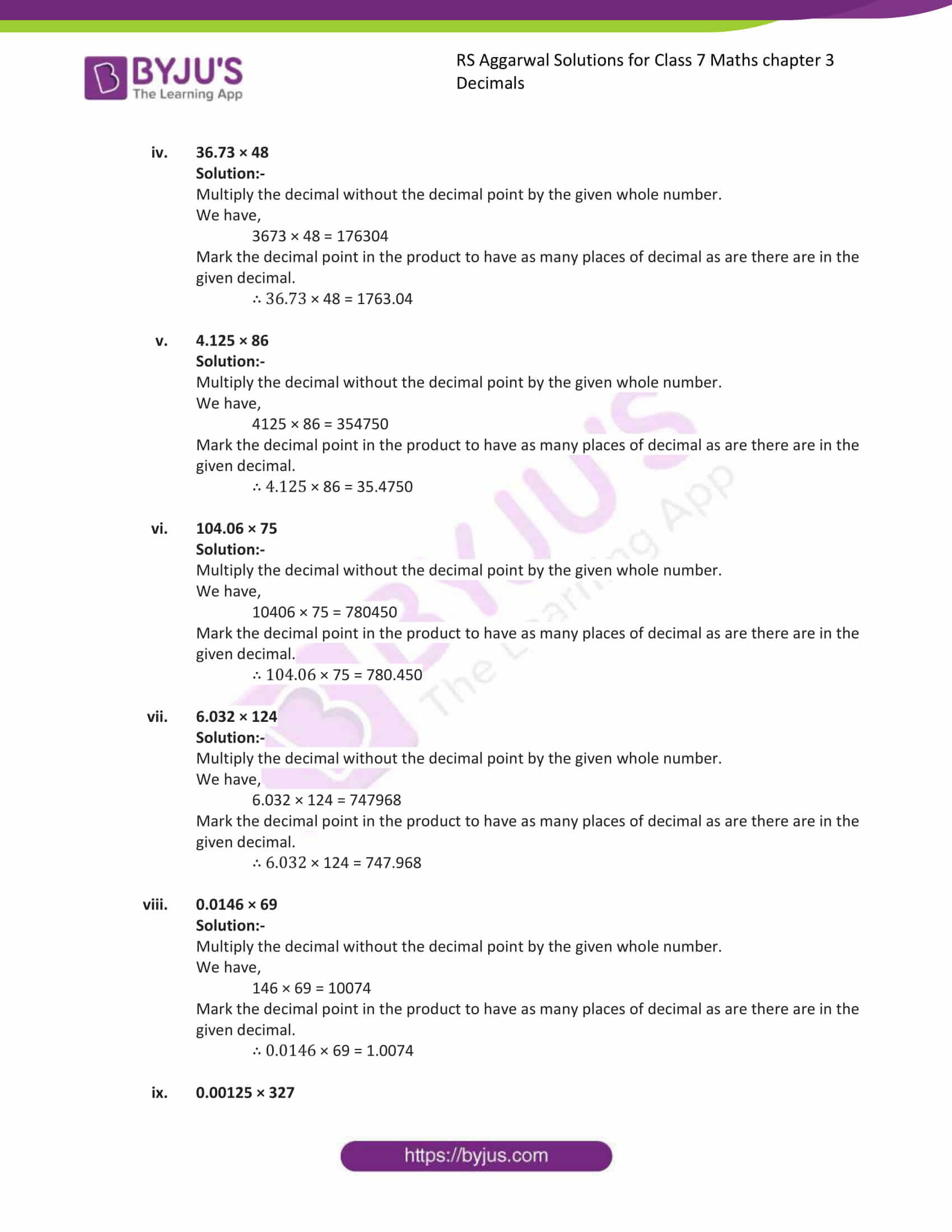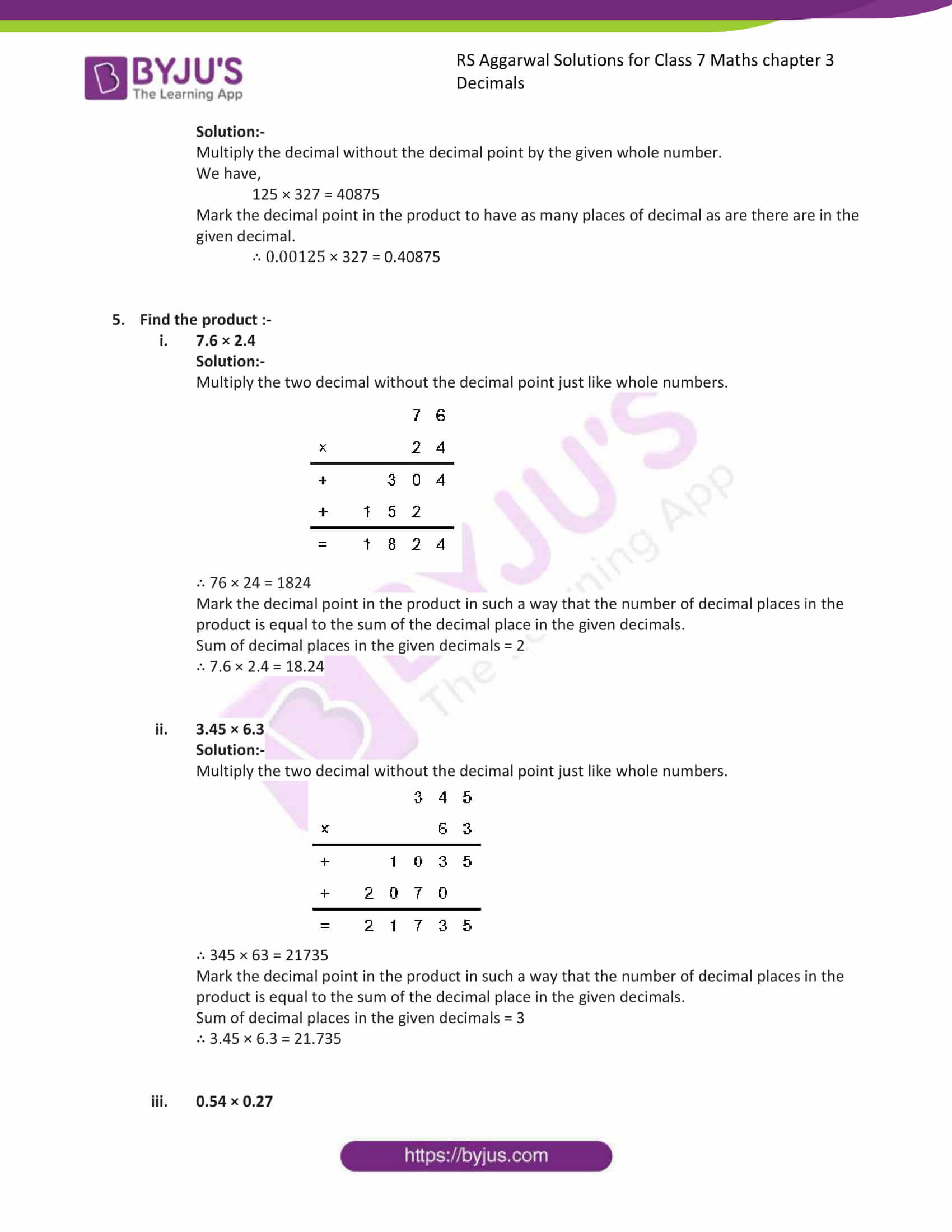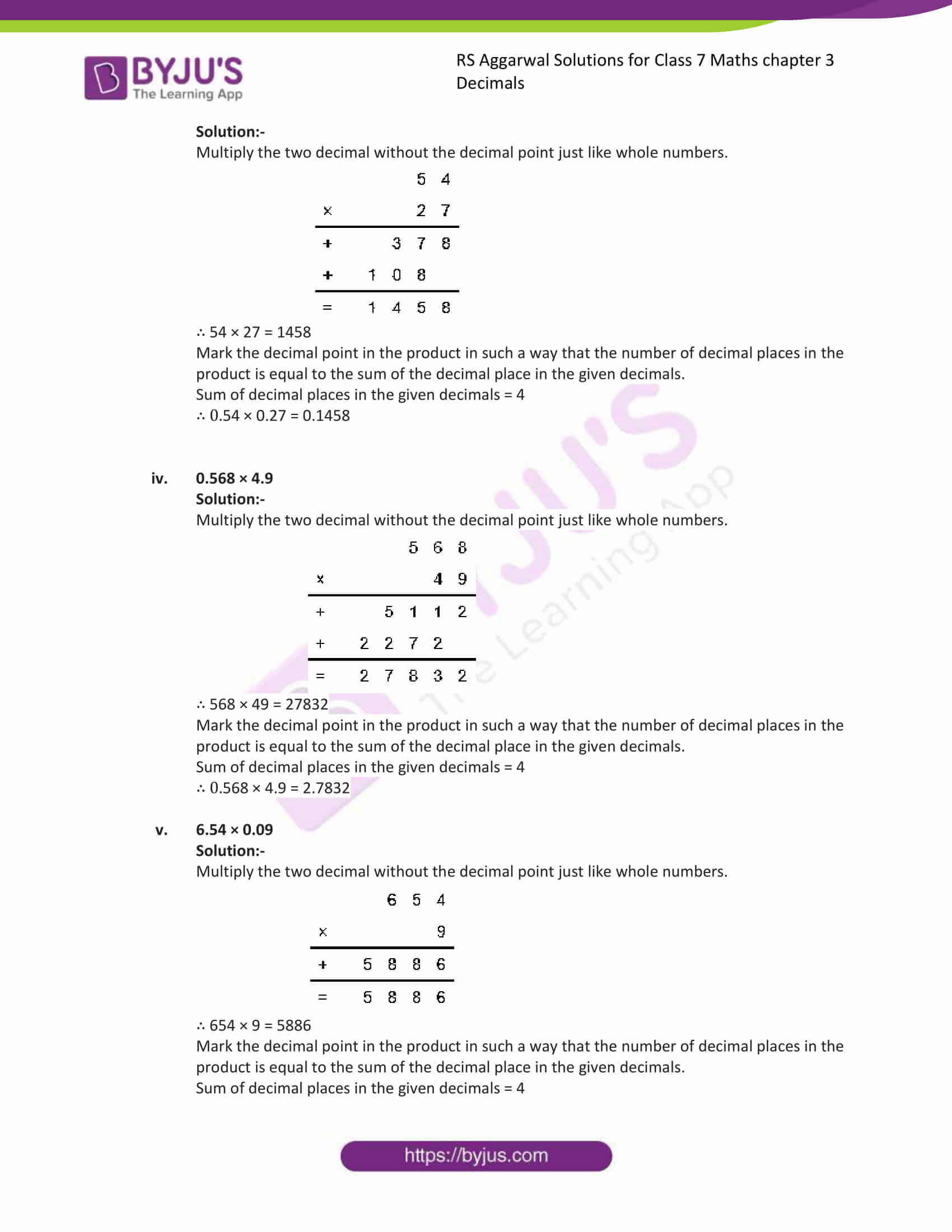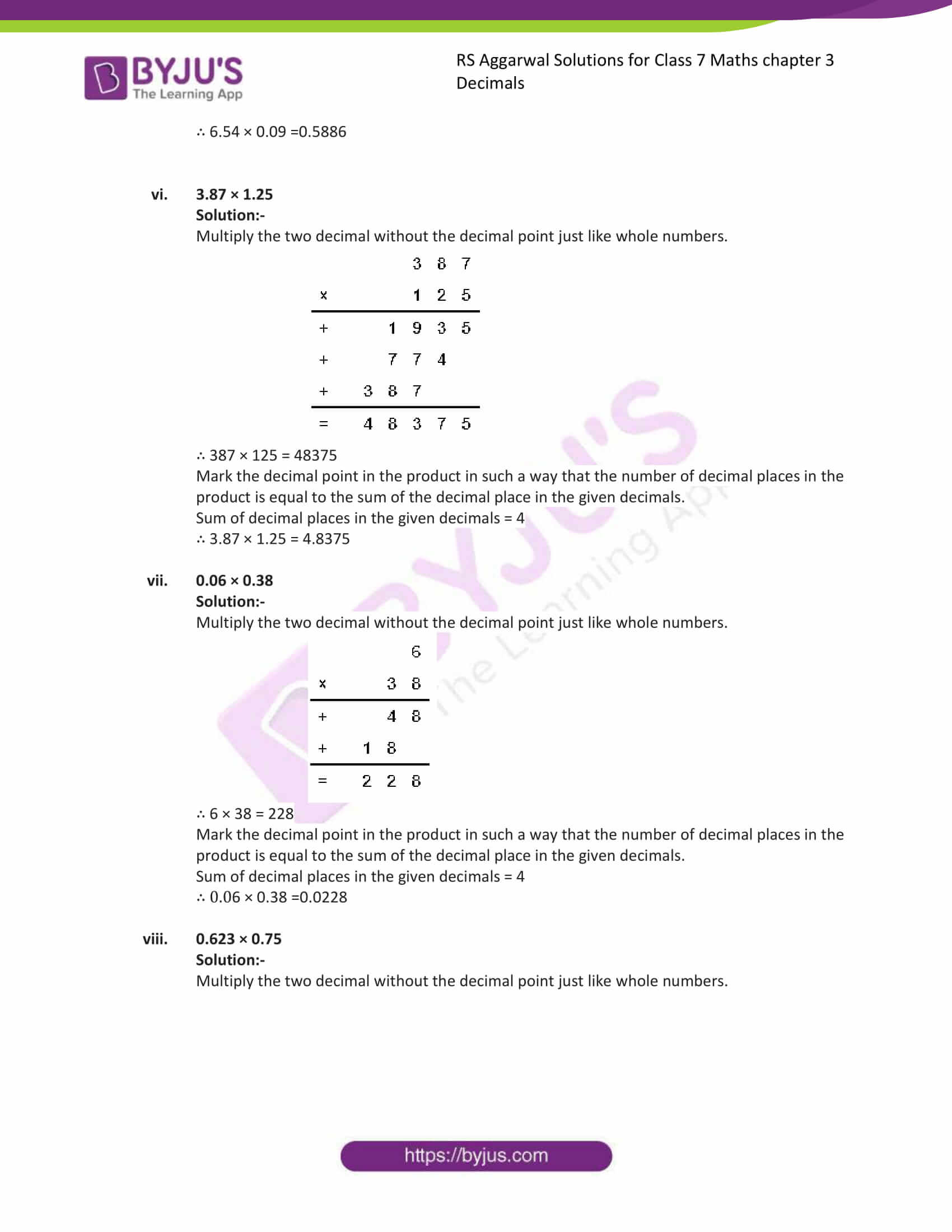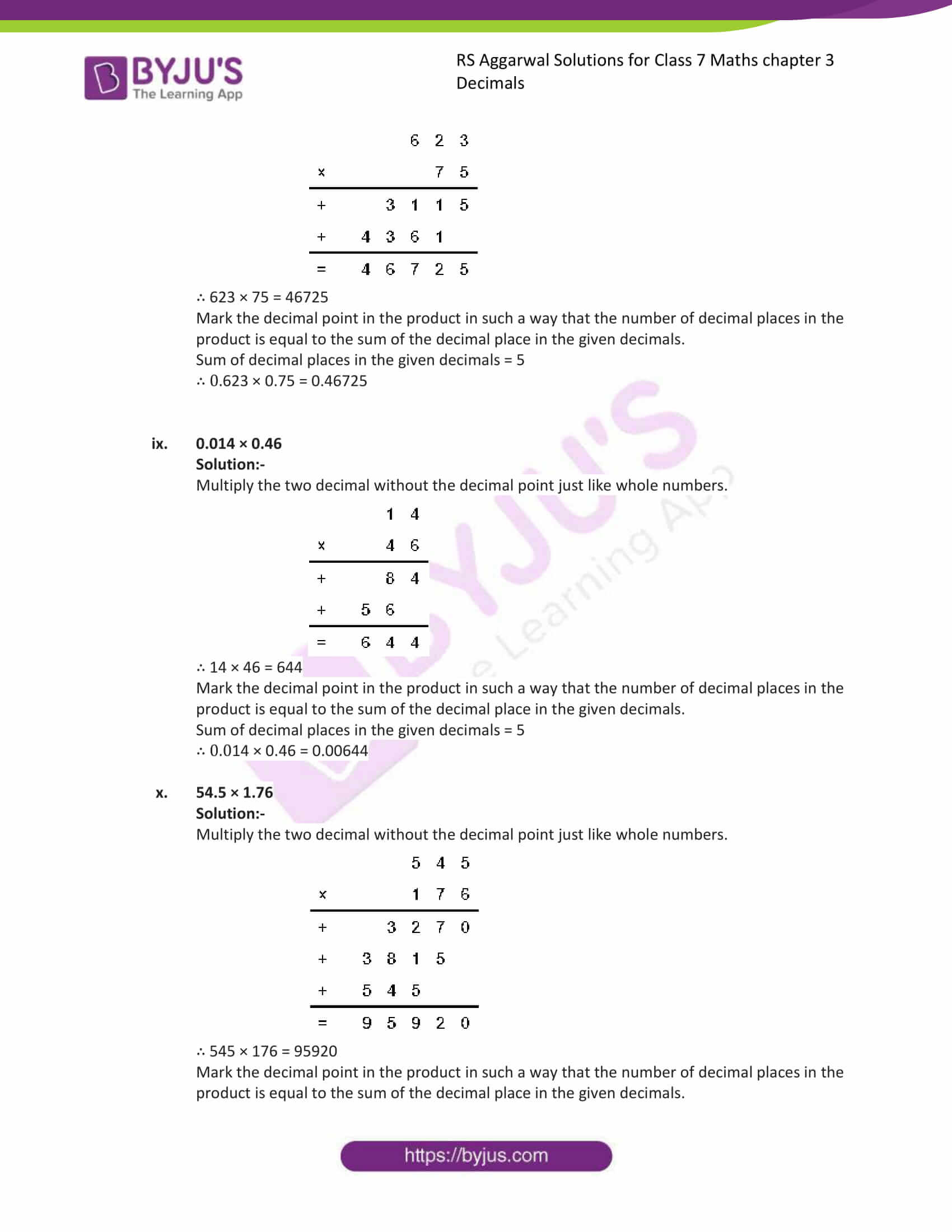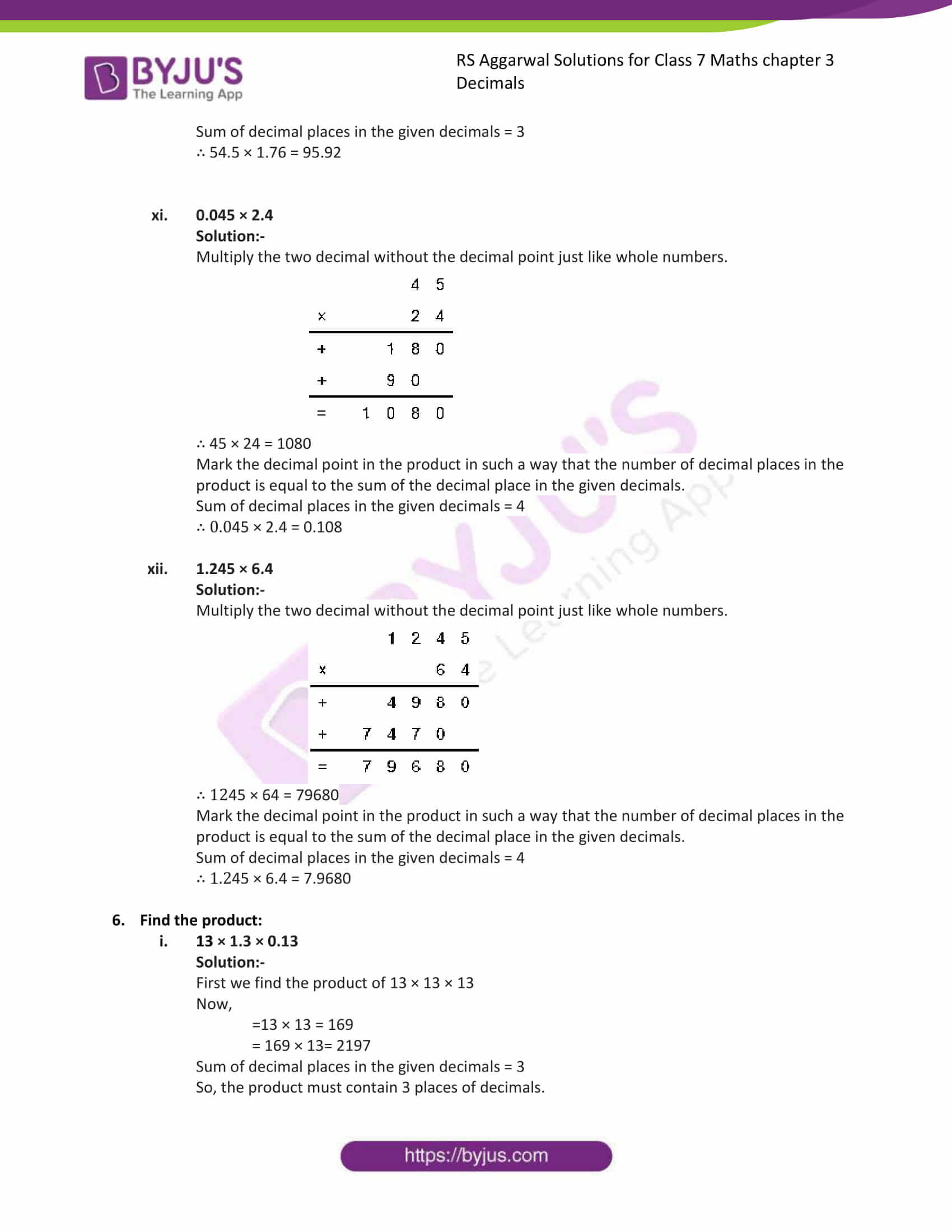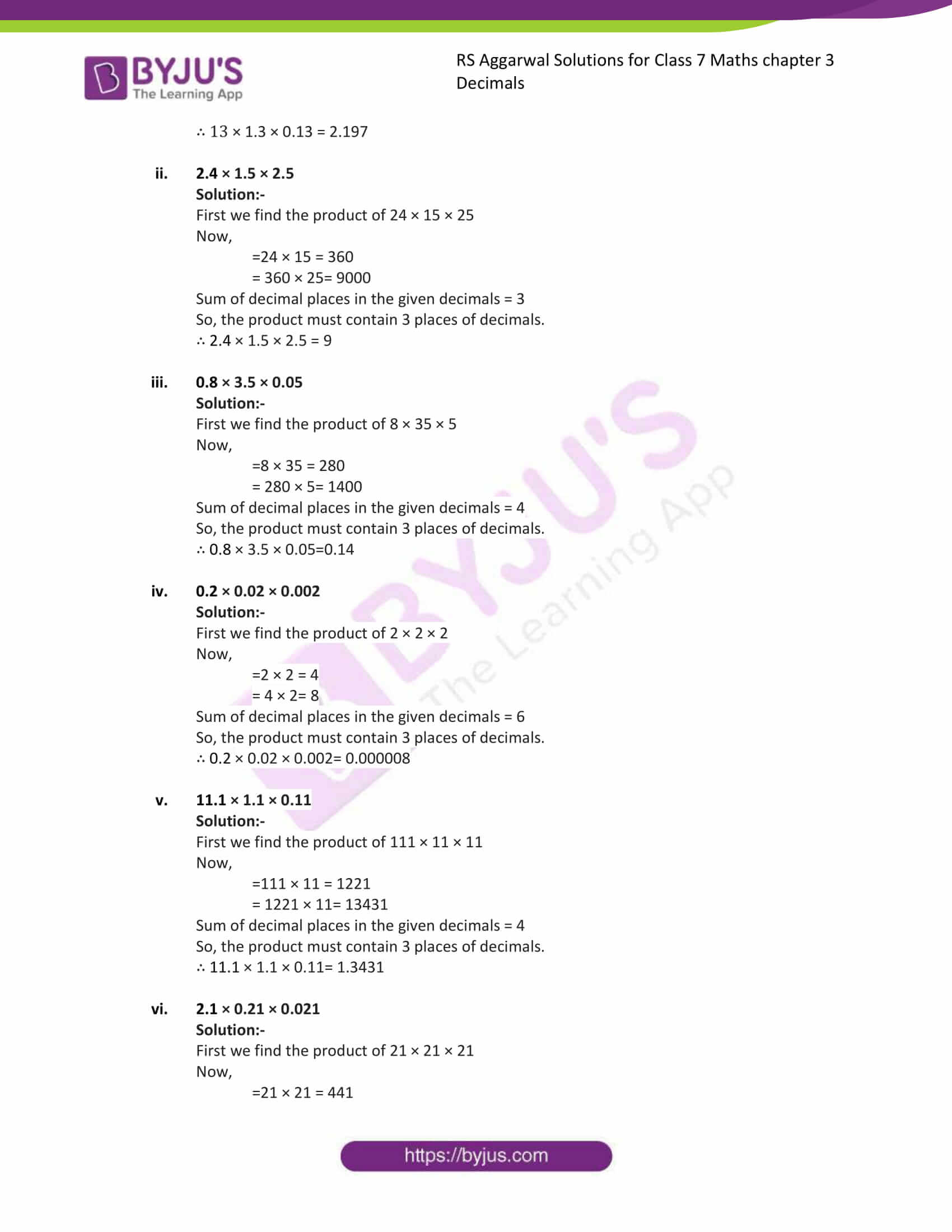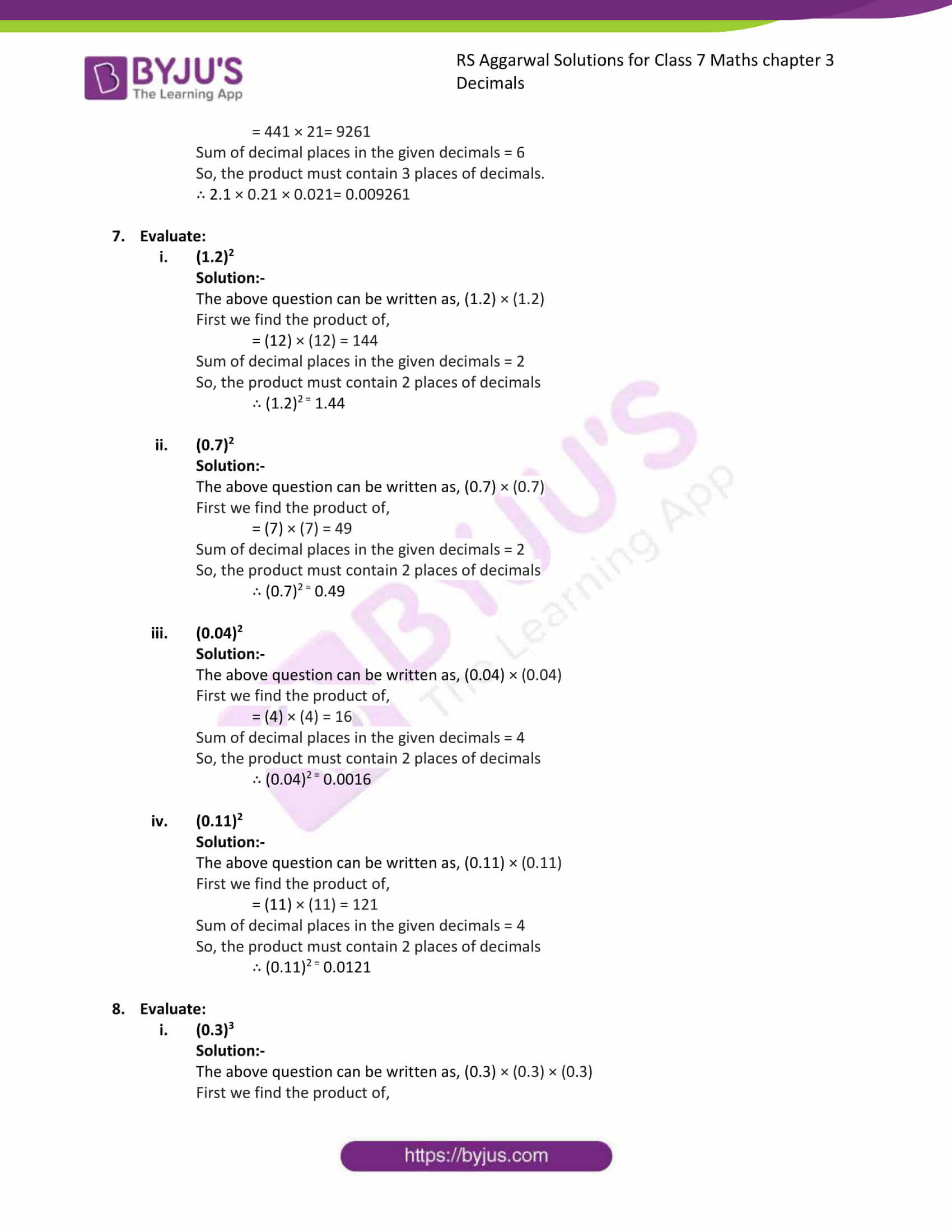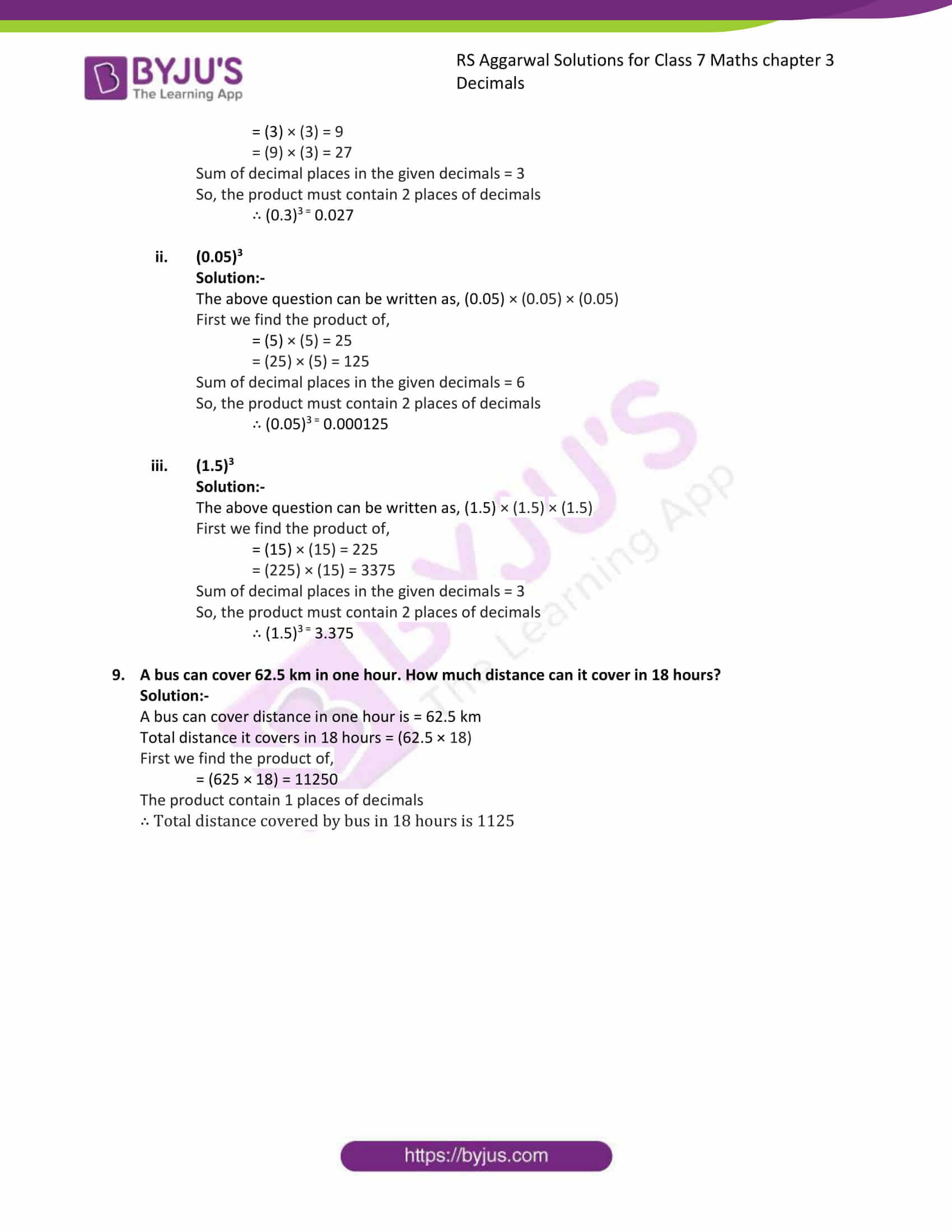### Access answers to Maths RS Aggarwal Solutions for Class 7 Chapter 3 – Decimals Exercise 3C

1. Find the product:

(i) 73.92 × 10

Solution:-

On multiplying a decimal by 10, the decimal point is shifted to the right by one place.

We have,

73.92 × 10 = 739.2

(ii) 7.54 × 10

Solution:-

On multiplying a decimal by 10, the decimal point is shifted to the right by one place.

We have,

7.54 × 10 = 75.4

(iii) 84.003 × 10

Solution:-

On multiplying a decimal by 10, the decimal point is shifted to the right by one place.

We have,

84.003 × 10 = 840.03

(iv) 0.83 × 10

Solution:-

On multiplying a decimal by 10, the decimal point is shifted to the right by one place.

We have,

0.83 × 10 = 8.3

(v) 0.7 × 10

Solution:-

On multiplying a decimal by 10, the decimal point is shifted to the right by one place.

We have,

0.7 × 10 = 7

(vi) 0.032 × 10

Solution:-

On multiplying a decimal by 10, the decimal point is shifted to the right by one place.

We have,

0.032 × 10 = 0.32

2. Find the product:

(i) 2.397 × 100

Solution:-

On multiplying a decimal by 100, the decimal point is shifted to the right by two places.

We have,

2.397 × 100 = 239.7

(ii) 6.83 × 100

Solution:-

On multiplying a decimal by 100, the decimal point is shifted to the right by two places.

We have,

6.83 × 100 = 683

(iii) 2.9 × 100

Solution:-

On multiplying a decimal by 100, the decimal point is shifted to the right by two places.

We have,

2.9 × 100 = 290

(iv) 0.08 × 100

Solution:-

On multiplying a decimal by 100, the decimal point is shifted to the right by two places.

We have,

0.08 × 100 = 8

(v) 0.6 × 100

Solution:-

On multiplying a decimal by 100, the decimal point is shifted to the right by two places.

We have,

0.6 × 100 = 60

(vi) 0.06 × 100

Solution:-

On multiplying a decimal by 100, the decimal point is shifted to the right by two places.

We have,

0.003 × 100 = 0.3

3. Find the product:

(i) 6.7314 × 1000

Solution:-

On multiplying a decimal by 1000, the decimal point is shifted to the right by three places.

We have,

6.7314 × 1000 = 6731.4

(ii) 0.182 × 1000

Solution:-

On multiplying a decimal by 1000, the decimal point is shifted to the right by three places.

We have,

0.182 × 1000 = 182

(iii) 0.076 × 1000

Solution:-

On multiplying a decimal by 1000, the decimal point is shifted to the right by three places.

We have,

0.076 × 1000 = 76

(iv) 6.25 × 1000

Solution:-

On multiplying a decimal by 1000, the decimal point is shifted to the right by three places.

We have,

6.25 × 1000 = 6250

(v) 4.8 × 1000

Solution:-

On multiplying a decimal by 1000, the decimal point is shifted to the right by three places.

We have,

4.8 × 1000 = 4800

(vi) 0.06 × 1000

Solution:-

On multiplying a decimal by 1000, the decimal point is shifted to the right by three places.

We have,

0.06 × 1000 = 60

4. Find the product:

(i) 5.4 × 16

Solution:-

Multiply the decimal without the decimal point by the given whole number.

We have,

54 × 16 = 864

Mark the decimal point in the product to have as many places of decimal as are there are in the given decimal.

∴ 5.4 × 16 = 8.64

(ii) 3.65 × 19

Solution:-

Multiply the decimal without the decimal point by the given whole number.

We have,

365 × 19 = 6935

Mark the decimal point in the product to have as many places of decimal as are there are in the given decimal.

∴ 3.65 × 19 = 69.35

(iii) 0.854 × 12

Solution:-

Multiply the decimal without the decimal point by the given whole number.

We have,

854 × 12 = 10248

Mark the decimal point in the product to have as many places of decimal as are there are in the given decimal.

∴ 0.854 × 12 = 10.248

(iv) 36.73 × 48

Solution:-

Multiply the decimal without the decimal point by the given whole number.

We have,

3673 × 48 = 176304

Mark the decimal point in the product to have as many places of decimal as are there are in the given decimal.

∴ 36.73 × 48 = 1763.04

(v) 4.125 × 86

Solution:-

Multiply the decimal without the decimal point by the given whole number.

We have,

4125 × 86 = 354750

Mark the decimal point in the product to have as many places of decimal as are there are in the given decimal.

∴ 4.125 × 86 = 35.4750

(vi) 104.06 × 75

Solution:-

Multiply the decimal without the decimal point by the given whole number.

We have,

10406 × 75 = 780450

Mark the decimal point in the product to have as many places of decimal as are there are in the given decimal.

∴ 104.06 × 75 = 780.450

(vii) 6.032 × 124

Solution:-

Multiply the decimal without the decimal point by the given whole number.

We have,

6.032 × 124 = 747968

Mark the decimal point in the product to have as many places of decimal as are there are in the given decimal.

∴ 6.032 × 124 = 747.968

(viii) 0.0146 × 69

Solution:-

Multiply the decimal without the decimal point by the given whole number.

We have,

146 × 69 = 10074

Mark the decimal point in the product to have as many places of decimal as are there are in the given decimal.

∴ 0.0146 × 69 = 1.0074

(ix) 0.00125 × 327

Solution:-

Multiply the decimal without the decimal point by the given whole number.

We have,

125 × 327 = 40875

Mark the decimal point in the product to have as many places of decimal as are there are in the given decimal.

∴ 0.00125 × 327 = 0.40875

5. Find the product :-

(i) 7.6 × 2.4

Solution:-

Multiply the two decimal without the decimal point just like whole numbers.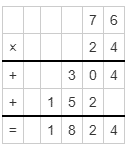∴ 76 × 24 = 1824

Mark the decimal point in the product in such a way that the number of decimal places in the product is equal to the sum of the decimal place in the given decimals.

Sum of decimal places in the given decimals = 2

∴ 7.6 × 2.4 = 18.24

(ii) 3.45 × 6.3

Solution:-

Multiply the two decimal without the decimal point just like whole numbers.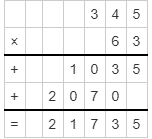∴ 345 × 63 = 21735

Mark the decimal point in the product in such a way that the number of decimal places in the product is equal to the sum of the decimal place in the given decimals.

Sum of decimal places in the given decimals = 3

∴ 3.45 × 6.3 = 21.735

(iii) 0.54 × 0.27

Solution:-

Multiply the two decimal without the decimal point just like whole numbers.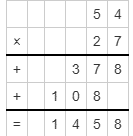∴ 54 × 27 = 1458

Mark the decimal point in the product in such a way that the number of decimal places in the product is equal to the sum of the decimal place in the given decimals.

Sum of decimal places in the given decimals = 4

∴ 0.54 × 0.27 = 0.1458

(iv) 0.568 × 4.9

Solution:-

Multiply the two decimal without the decimal point just like whole numbers.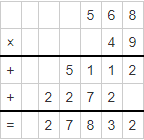∴ 568 × 49 = 27832

Mark the decimal point in the product in such a way that the number of decimal places in the product is equal to the sum of the decimal place in the given decimals.

Sum of decimal places in the given decimals = 4

∴ 0.568 × 4.9 = 2.7832

(v) 6.54 × 0.09

Solution:-

Multiply the two decimal without the decimal point just like whole numbers.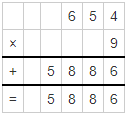∴ 654 × 9 = 5886

Mark the decimal point in the product in such a way that the number of decimal places in the product is equal to the sum of the decimal place in the given decimals.

Sum of decimal places in the given decimals = 4

∴ 6.54 × 0.09 =0.5886

(vi) 3.87 × 1.25

Solution:-

Multiply the two decimal without the decimal point just like whole numbers.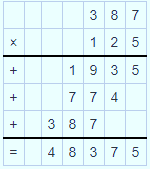∴ 387 × 125 = 48375

Mark the decimal point in the product in such a way that the number of decimal places in the product is equal to the sum of the decimal place in the given decimals.

Sum of decimal places in the given decimals = 4

∴ 3.87 × 1.25 = 4.8375

(vii) 0.06 × 0.38

Solution:-

Multiply the two decimal without the decimal point just like whole numbers.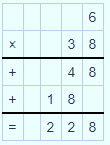∴ 6 × 38 = 228

Mark the decimal point in the product in such a way that the number of decimal places in the product is equal to the sum of the decimal place in the given decimals.

Sum of decimal places in the given decimals = 4

∴ 0.06 × 0.38 =0.0228

(viii) 0.623 × 0.75

Solution:-

Multiply the two decimal without the decimal point just like whole numbers.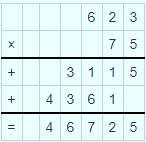∴ 623 × 75 = 46725

Mark the decimal point in the product in such a way that the number of decimal places in the product is equal to the sum of the decimal place in the given decimals.

Sum of decimal places in the given decimals = 5

∴ 0.623 × 0.75 = 0.46725

(ix) 0.014 × 0.46

Solution:-

Multiply the two decimal without the decimal point just like whole numbers.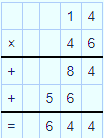∴ 14 × 46 = 644

Mark the decimal point in the product in such a way that the number of decimal places in the product is equal to the sum of the decimal place in the given decimals.

Sum of decimal places in the given decimals = 5

∴ 0.014 × 0.46 = 0.00644

(x) 54.5 × 1.76

Solution:-

Multiply the two decimal without the decimal point just like whole numbers.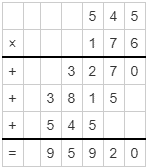∴ 545 × 176 = 95920

Mark the decimal point in the product in such a way that the number of decimal places in the product is equal to the sum of the decimal place in the given decimals.

Sum of decimal places in the given decimals = 3

∴ 54.5 × 1.76 = 95.92

(xi) 0.045 × 2.4

Solution:-

Multiply the two decimal without the decimal point just like whole numbers.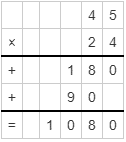∴ 45 × 24 = 1080

Mark the decimal point in the product in such a way that the number of decimal places in the product is equal to the sum of the decimal place in the given decimals.

Sum of decimal places in the given decimals = 4

∴ 0.045 × 2.4 = 0.108

(xii) 1.245 × 6.4

Solution:-

Multiply the two decimal without the decimal point just like whole numbers.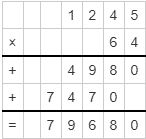∴ 1245 × 64 = 79680

Mark the decimal point in the product in such a way that the number of decimal places in the product is equal to the sum of the decimal place in the given decimals.

Sum of decimal places in the given decimals = 4

∴ 1.245 × 6.4 = 7.9680

6. Find the product:

(i) 13 × 1.3 × 0.13

Solution:-

First we find the product of 13 × 13 × 13

Now,

=13 × 13 = 169

= 169 × 13= 2197

Sum of decimal places in the given decimals = 3

So, the product must contain 3 places of decimals.

∴ 13 × 1.3 × 0.13 = 2.197

(ii) 2.4 × 1.5 × 2.5

Solution:-

First we find the product of 24 × 15 × 25

Now,

=24 × 15 = 360

= 360 × 25= 9000

Sum of decimal places in the given decimals = 3

So, the product must contain 3 places of decimals.

∴ 2.4 × 1.5 × 2.5 = 9

(iii) 0.8 × 3.5 × 0.05

Solution:-

First we find the product of 8 × 35 × 5

Now,

=8 × 35 = 280

= 280 × 5= 1400

Sum of decimal places in the given decimals = 4

So, the product must contain 3 places of decimals.

∴ 0.8 × 3.5 × 0.05=0.14

(iv) 0.2 × 0.02 × 0.002

Solution:-

First we find the product of 2 × 2 × 2

Now,

=2 × 2 = 4

= 4 × 2= 8

Sum of decimal places in the given decimals = 6

So, the product must contain 3 places of decimals.

∴ 0.2 × 0.02 × 0.002= 0.000008

(v) 11.1 × 1.1 × 0.11

Solution:-

First we find the product of 111 × 11 × 11

Now,

=111 × 11 = 1221

= 1221 × 11= 13431

Sum of decimal places in the given decimals = 4

So, the product must contain 3 places of decimals.

∴ 11.1 × 1.1 × 0.11= 1.3431

(vi) 2.1 × 0.21 × 0.021

Solution:-

First we find the product of 21 × 21 × 21

Now,

=21 × 21 = 441

= 441 × 21= 9261

Sum of decimal places in the given decimals = 6

So, the product must contain 3 places of decimals.

∴ 2.1 × 0.21 × 0.021= 0.009261

7. Evaluate:

(i) (1.2)2

Solution:-

The above question can be written as, (1.2) × (1.2)

First we find the product of,

= (12) × (12) = 144

Sum of decimal places in the given decimals = 2

So, the product must contain 2 places of decimals

∴ (1.2)2 = 1.44

(ii) (0.7)2

Solution:-

The above question can be written as, (0.7) × (0.7)

First we find the product of,

= (7) × (7) = 49

Sum of decimal places in the given decimals = 2

So, the product must contain 2 places of decimals

∴ (0.7)2 = 0.49

(iii) (0.04)2

Solution:-

The above question can be written as, (0.04) × (0.04)

First we find the product of,

= (4) × (4) = 16

Sum of decimal places in the given decimals = 4

So, the product must contain 2 places of decimals

∴ (0.04)2 = 0.0016

(iv) (0.11)2

Solution:-

The above question can be written as, (0.11) × (0.11)

First we find the product of,

= (11) × (11) = 121

Sum of decimal places in the given decimals = 4

So, the product must contain 2 places of decimals

∴ (0.11)2 = 0.0121

8. Evaluate:

(i) (0.3)3

Solution:-

The above question can be written as, (0.3) × (0.3) × (0.3)

First we find the product of,

= (3) × (3) = 9

= (9) × (3) = 27

Sum of decimal places in the given decimals = 3

So, the product must contain 2 places of decimals

∴ (0.3)3 = 0.027

(ii) (0.05)3

Solution:-

The above question can be written as, (0.05) × (0.05) × (0.05)

First we find the product of,

= (5) × (5) = 25

= (25) × (5) = 125

Sum of decimal places in the given decimals = 6

So, the product must contain 2 places of decimals

∴ (0.05)3 = 0.000125

(iii) (1.5)3

Solution:-

The above question can be written as, (1.5) × (1.5) × (1.5)

First we find the product of,

= (15) × (15) = 225

= (225) × (15) = 3375

Sum of decimal places in the given decimals = 3

So, the product must contain 2 places of decimals

∴ (1.5)3 = 3.375

9. A bus can cover 62.5 km in one hour. How much distance can it cover in 18 hours?

Solution:-

A bus can cover distance in one hour is = 62.5 km

Total distance it covers in 18 hours = (62.5 × 18)

First we find the product of,

= (625 × 18) = 11250

The product contain 1 places of decimals

∴ Total distance covered by bus in 18 hours is 1125

### Access other exercises of RS Aggarwal Solutions For Class 7 Chapter 3 – Decimals

Exercise 3E Solutions